# CAN A MACHINE REPLICATE THE HUMAN BRAIN?

Written by
David Silverstein & Suzette Lyn

Introduction

In today’s day and age the words artificial intelligence divide people into two groups.  One group is either excited about where this will take us, and the other group associates this with the stigma and fear that machines will one day take over mankind.

Of course the origin of ideas basically came from science fiction movies such as “Terminator” and books like “I, Robot”, where machines learned to think independently without any commands from their creators, which followed by the machines taking over humanity.

To many, such stories have instilled fear that  a machine can learn to think like a human. But Can a machine really replicate the human brain?

The Rise of the Machine

The rapid rise of artificial intelligence and machine learning has resulted in massive leaps in technological advancement.  We have continuously seen artificial intelligence making significantly increases in processing speed in addition to being able to carry out more complex tasks.  In today’s day and age we are seeing more people becoming more dependent on computers and artificial intelligence.  This is especially true when it comes to coding and simulations in addition to working out extremely long and complex equations.  There are certain equations that could take a human several hours or even several days to be able to work out, while a computer would in principle have the solution in a matter of minutes or less.  We continue to see specific programming packages made specifically with the sole purpose to be able to carry out very particular and complex computations.  While there may not be a specific programming package that is able to carry out a certain type of computation, it should only be a matter of time before one is made.  Aside from the specific programming packages, we see different coding languages and different programs that are tailor made for very specific purposes whether this be something like Java, Mathematica, Python, Matlab, C++ etc.

Yet despite all these remarkable achievements, this begs the question if machines will replace humans, or more specifically if a machine can completely replicate a human brain.  There has been a lot of debate from those who think a machine can have a brain and those who don’t.  This includes those who do work in computer science, neuroscience, philosophy, physics and mathematics.  All sides have made very valid points, yet ultimately there is one missing link that both sides and all disciplines believe needs to be understood better.  That missing link happens to be consciousness which will be discussed in more detail later on.  Coming from the perspective of those who don’t think a machine can replicate the human brain (or against the A.I.) there have been both naive and very sophisticated arguments as well as mathematical modeling that has been done.

A Historical Perspective

To examine such a question more carefully, one has to look at the roots of the problem and some of the first questions that were asked.  It was in 1936 that Alan Turing first introduced what is called the Turing machine which contains a long string of numbers which have the task of carrying out inputs and outputs.  Despite how simple it is, a Turing machine is able to stimulate any computer’s algorithmic logic.  In other words any mechanical set of rules put forward for calculations and problem solving could be stimulated by a Turing machine.  The Turning machine can in fact be generalized to the general purpose computer.  It has been speculated that Alan Turing had suspensions and asked himself the question if the brain is in fact a Turing machine, or does it go beyond it.  In 1951 there was a famous quote by Turing which stated the following “If it is accepted that real brains are a type of machine it will follow that our digital computer suitably programmed will behave like a brain”.  It should be noted that such an argument requires many assumptions that can easily be challenged.  Such statements have been put forward stating that this type of machine should in principle be predictable by calculation.  Yet it was put forward by Sir Aruthor Eddington that due to the indeterminacy of quantum mechanics that no such prediction should be theoretically possible.

Turing himself also realized that there appears to be a lot of indeterminacy surrounding the human brain, something which is indeed very reminiscent of quantum mechanics.  Due to quantum mechanics having lots of random elements, Turing realized that if a Turing machine tried to replicate quantum mechanics, it would no longer be a Turing machine which is made to be predictable and deterministic.  So immediately there were already speculations involving the challenges facing such a question.  Other interesting statements that have been made at the time involved one of the following statements “If we wish to imitate anything so complicated as the human brain we need a much larger machine than any of the computers at present available.  We probably need something 100x as large as the Manchester computer”.  At the time the Manchester computer was the largest and most powerful computer and something at least 100x larger or significantly more powerful was suspected to be needed in order to replicate the human brain.  Modern day computers are significantly more powerful and are able to carry out tasks that the manchester computer would never be able to achieve.  Yet if the brain can be replicated by a computer; to this day is still highly debatable.

Beyond the Turing Machine

Other than the thought and paradoxical task of designing a Turing machine that would allow indeterministic outcomes, there were more sophisticated arguments that began to be put forward.  One such argument by Turing involved ordinal and cardinal numbers.  Arguments of this type that were put forward by Turing were faced with asking about the perception of mathematical truth.  In other words, how do we judge if a mathematical statement is true or false.  Before we take a look at ordinal numbers, let us begin by looking at an easier example involving natural numbers, 0, 1, 2, 3, 4 etc.  Yet before jumping into some examples, the logic here is that the perception of mathematical truth cannot be reduced to a set of mechanical rules.  This comes from a famous theorem that was put forward by the great mathematician and logician Kurt Godel.  Turing himself was very familiar with this theorem which he included in his 1936 paper due to the fact that he was very concerned with things that computers couldn’t do.  To paraphrase what Godel’s theorem says, the following will be said:

Godel’s Theorem tells us that for any set of mechanical theorem proving rules R, we can construct a mathematical state G(R) which, if we believe in the validity of R, we must accept as true; yet G(R) cannot be proven using R alone.

What we mean by any mechanical theorem proving rules, we mean rules that can be checked by a computer.  Therefore if you follow these rules and you come to a conclusion that it proves some mathematical statement of the kind that is being discussed, then that alleged proof can be checked by a computer.  From there one can construct a particular mathematical statement which we call G(R).  Therefore if one trusts the rules, one can conclude that G(R) is a true statement.  However, it cannot be trusted simply by using the rules of R, and we must see what are the rules of R before one can trust the rules.  While this may sound contradictory, what it is really saying is that we cannot just immediately accept the rules of R as true.  One needs to carefully examine the rules before we can determine that the rules are trustworthy.  In other words we cannot simply restrict ourselves to a very specific set of rules and if one does, one may have to accept them as being true regardless of what the rules state.  Yet what is very striking about this statement is that the belief and choice one makes to determine if the rules are trustworthy is something that already extends beyond the rules of R.  Therefore beyond simple mathematical induction.  Naturally one may now be thinking what would be an example of such rules that are being discussed.  Therefore some examples will now be discussed.

Let us begin with an example of the natural numbers:  n = 1, 2, 3, 4…

We wish to prove that some property P(n) is true for all natural numbers n

1).  Establish P(0)

2).  Show that the truth of P(n + 1) follows from the truth of P(n).

What is being asked here is if we start off from the property P(0) can we write down all the natural numbers?  Well if we insert zero into P(0) we certainly get zero since P(0) = 0, but what about the other natural numbers.  That is when we establish P(n + 1), we begin with n = 0:  P(0 +1) = 1.  Moving onto n = 1 we get P(1 + 1) = 2, for n = 2 we get P(2 + 1) = 3, for n = 3 we get 4, n = 4 we get 5 etc.  However, in order to write down all of the natural numbers it would take an infinite amount of time due to there being an infinite amount of natural numbers.  Yet one has to ask themselves the question if using Peano arithmetic (arithmetic that focuses on the real numbers) is enough to satisfy the computational rules?  Godel’s theorem states that the answer is no.

Once again it would be best to provide an example of something that cannot be proved using simple mathematical induction which in our case was with the Peano axioms.  One such example that was presented by the English mathematical physicist Sir Roger Penrose, would be the game Hercules and the Hydra that was due to the work of two mathematicians, Laurence Kirby and Jeffery Paris in 1982.  In this game just like in the Greek tale, Hercules battles a hydra.  In this game we need to establish that Hercules is incredibly strong, never gives up, has unlimited stamina yet isn’t the brightest of people.  Hercules cuts off one of the heads of the Hydra, yet whenever he cuts a head off of the hydra, the hydra grows more heads.  Therefore, he repeats the same process without ever stopping and more heads continue to grow.  So one has to ask the question if he is going to win, or will he just continue chopping off heads forever?  What is remarkable about the theorem surrounding this by Kirby and Paris, is that no matter how foolish Hercules is, he is going to always win.  To prove this we need to look at the properties of the ordinal numbers that I very briefly mentioned before.  In the game of Hercules and the Hydra, the heads of the hydra represent ordinal numbers and each time a head is chopped off it makes a smaller ordinal number and as the ordinal numbers get smaller and smaller they will eventually terminate and he will win.  However, it takes an incredible length of time, and in some cases longer than the age of the universe.  While this is a famous example, it would be best if I were to give an example of how ordinal numbers are written down.  Ordinal numbers represent what is known as a hereditary base notation.  It is customary that ordinal numbers are written down in lower case omegas.  From here we can write out the ordinal numbers in hereditary base notation in the following way: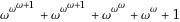.  In order to make things more transparent we can now substitute our omegas with real numbers using a theorem that was put forward by the mathematician Ruben Goodstein.  It should be noted that Goodstein’s theorem is a Godel-like theorem that is just written down in hereditary base notation using ordinal numbers.  Using the information we now have, we are going to present the theorem in detail.

Here we have our ordinal numbers.  From here we are going to substitute our omegas with positive whole numbers and we will get the following number.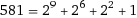.  Of course we can break this down further by writing the exponents using the same hereditary base notation since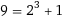,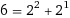and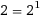.  So we can now write 581 as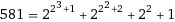We can still break this down further since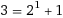So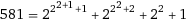From here we are now going to apply a succession of simple operations for the expression above.

A).  Increase the base by 1

B).  Subtract by 1

The bases in the above expression are the 2’s, so since we need to increase the bases by 1, and are going to change all of our 2’s into 3’s.  So we will now get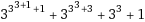Now we are going to apply (b) by subtracting by 1 and we get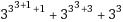Apply (a) to obtain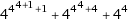applying rule (b) now yields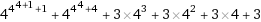Applying operation (a) we then get the following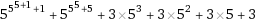and then apply operation (b) which gives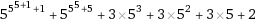We now continue this sequence going from (a), (b), (a), (b), (a), (b) continuously.  We see the numbers continue to get significantly larger as we continue, and one may think that the numbers would only get larger and larger.  However, this is not so since Goodstein’s theorem tells us that no matter how large the numbers, they will eventually go to zero.  To show this we can start off withApply operation (a) we get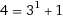Applying operation (b) we get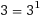Now apply (a) and we have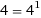Since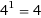it would be equivalent to write it as 4 = 4.  We now have no base to increase and operation (a) will not affect our sequence and only operation (b) will.  We then get 3 = 3,  2 = 2,  1 = 1 and 0 = 0.  We have now shown that Goodstein’s theorem will indeed force our numbers to eventually be equal to zero.

We must remind ourselves that Goodstein’s theorem is a Godel-like theorem.  We have our mechanical theorem proven rules R and we were able to construct a mathematical statement G(R).  This was just like our first example we used when we had all the natural n = 1, 2, 3, 4, and we had the property P(n).  From there we began with P(0) and moved onto P(n + 1).  We showed that this is indeed a trusted algorithmic procedure which would in principle be able to include all the natural numbers despite the fact that it would take an infinite amount of time.  Yet, when we moved onto Goodstein’s theorem using the ordinal numbers we have shown that this is not so.  Despite the fact that it is a Godel-like theorem, we cannot include all the natural numbers, and when we think the numbers appear to be following the same endless sequence of getting larger and larger but eventually they end up going to zero.  This goes to show that we cannot use simple mathematical induction of mechanical proven rules to be able to show the validity of Goodstein’s theorem.

It should of course be stated that there are arguments against Godel-like theorems.  One such argument is known as the Errors Argument.  This was an argument stated by Turing who at some point eventually thought that perhaps we are a type of computational system.  The errors argument states:  Human mathematicians make errors, so rigorous Godel-type arguments do not apply.  Turing gave a lecture at the mathematical society in 1947 where he quoted the following “If a machine is expected to be infallible, it cannot also be intelligent.  There are several theorems that state exactly that, but these theorems say nothing about how much intelligence may be displayed if a machine makes no pretence of infallibility.”  Hence something has to be able to make mistakes to be as intelligent as we are.  Counter arguments by those such as Penrose have been presented with Penrose stating how this seems like an implausible  let-out; and human mathematical errors are something that are able to be corrected by others or the same human.  Other arguments against Godel-type theorems would include the Extreme Complication Argument. This argument states that algorithms governing human mathematical understanding are so vastly complicated that their Godel statements are completely beyond reach.  Lastly there is a let-out by Godel himself which is rather ironic since he is in support that the human brain goes beyond the computational ability of a computer.  This argument is known as the Ignorance Of The Algorithm Argument which states:  We do not know the algorithmic process underlying our mathematical understanding, so we cannot construct it’s Godel statement.  He is saying that if we don’t know what R is we cannot construct G(R) and our Godel-type theorem cannot be constructed.  Yet this does raise the question; if one is building computers, in a sense one would know how they operate.  So does this ignorance apply to devices that one constructs themselves?  There have been other questions that have been asked by those such as Penrose and others, wondering; if we are some sort of unknown type of algorithm; how did this unknown algorithmic development come about?  It appears that the general consensus among him and other mathematicians, physicists and neuroscientists is what was briefly mentioned before, which happens to be consciousness.  Yet due to consciousness being something that is still very mysterious, how would we go about defining consciousness?

To better understand and define consciousness, there seems to be preliminaries one has to think about before defining and understanding consciousness.  It would seem natural that for something to be conscious, that entity first has to have intelligence.  From intelligence there also has to be understanding and from understanding there should then be awareness.  Of course all of these seem to fit together since it would be hard to say that an entity has intelligence if it isn’t able to understand.  Likewise it would appear very odd to state that an entity is able to have understanding if it doesn’t have awareness.  Finally from awareness it would appear that one would be able to go to the next level which is consciousness.  Tying everything together, I suppose one should ask the question: is a computer capable of genuine understanding and awareness?  If this were so it would seem that a computer may be able to be a conscious apparatus.  If not it would seem that a computer cannot replicate the human brain.  Before jumping directly at the heart of that question, how would one go about testing a computer to see if it is capable of genuine understanding and awareness?  Quotes by logicians such as Penrose have stated the following. “If understanding can be shown to be beyond computation, then intelligence is not a matter of computation; moreover certain aspects of awareness (perhaps all are) are beyond computation also.”  There have been many tests that have been put forward.  These tests that have been put forward are known as the Turing tests.

A Turing test is a test that is used to determine if a computer is able to think like a human.  A good example would be if a computer and human were to be paired together and would be hidden from the view of the interrogator.  The interrogator would then try to decide which one is the human and which one is the computer by simply questioning the two. It is important to know that the interrogator is not allowed to know any information about either the human or the computer before questioning the two.  The human answers the questions truthfully and has to convince the interrogator that they are indeed the human.  On the other hand the computer is programmed to “lie” and try to convince the interrogator that they are a human.  If the interrogator is unable to distinguish the difference between the human and the computer, then the computer has passed the Turing test.  The results of the Turing tests so far have been negative and a computer still has yet to pass the Turing test.  This has so far advocated that a computer has shown that it has no genuine understanding and awareness.  Yet, would a Turing test be something that is even enough to answer the question?  An example where the Turing test wouldn’t seem to be enough would be something like the Chinese Room argument which was put forward by the philosopher John Searle.  The argument states the following:  Searle imagines himself alone in a room where Chinese characters are being slipped under the door to him.  Searle is following a computer program where he is responding to Chinese characters, yet Searle himself knows nothing about any of the Chinese languages, yet he follows the program for manipulating symbols just like the computer where he sends strings of Chinese symbols under the door.  This leads those outside to suppose that there is a native Chinese speaker in the room.  The conclusion of the argument is that the programming of the computer may make it appear to understand the language, yet it is not able to produce real understanding.  Hence the Turing Test would be inadequate despite that it should be the first test that a computer should pass before looking at more complicated situations such as Searle’s Chinese room.

With tests like Turing tests and examples like Hercules and the Hydra as well as the Chinese room, it would seem that a computer really does lack true understanding and awareness.  Yet, so far I have not spoken or shown any actual proofs that a machine cannot replicate the human brain.  One would most likely be very curious to know and would ask the question if there are any actual proofs that were put forward addressing this question?  The answer is indeed yes!  This proof is actually a proof of Godel’s theorem.  Before jumping straight into the proof it would be useful to go over some of the notions that are used in the proof.  Recall our example using simple mathematical induction using Peano arithmetic when we listed all the natural numbers such as n = 1, 2, 3, 4 etc.  We had the function P(n) which contained all the natural numbers.  We then began to establish P(0) which equaled zero and then we established P(n + 1) which followed from the truth of P(n).  This truth allowed us to be able to in principle list all the natural numbers.  This type of formalism has a more technical name to it, which is called a Pi-1 sentence which is an assertion that some specific Turing machine action never terminates.  This is just like an algorithm or algorithmic procedure which is a string of zeros and ones that goes on indefinitely.  Since the proof of Godel’s theorem uses a Turing-like procedure such as Pi-1 sentences, the proof became known as the Godel-Turing theorem.  With all that said, the proof of the theorem is now going to be presented.

Godel’s theorem tells us that, for any set of mechanical theorem-proving statement rules R, we can construct a mathematical statement G(R) which if we believe in the validity of R, we must accept as true yet G(R) cannot be proved using R alone. R is a trusted algorithmic procedure for demonstrating certain Pi-1 sentences R(c,x) = true implies C(x) = infinity.  Proof 1).  Modify R to E, so that still E(c,x) = True implies C(x) = infinity but where we demand E(c,x) = false doesn’t even occur simply by putting E into a loop whenever R = false.  Proof 2).  Put x = c, so E(c,c) = true implies C(c) = infinity.  Proof 3).  E(x,x) is a computation depending on a single parameter, so it’s in Godel’s list, say at h, whence E(c,c) = H(c) where H(x) is the hth computation in Godel’s list, so putting c = h, E(h,h) = H(h) and taking c to be given by c = h we have c = h, so E(h,h) = true implies H(h) = infinity and then tells us that E(h,h) = infinity while H(h) = infinity.  Thus E can’t access this trusted conclusion!

That is the proof of Godel’s theorem and the proof is also known as the Godel-Turing theorem.  Yet what is this proof actually explicitly saying?  Well we have a list of different Pi-1 sentences and we are going to have to see which ones are true.  So how do we, or how does a machine possibly distinguish this?  We begin by talking about Godel’s theorem which we have spoken about.  We have our mechanical proven theorem rules R and we construct a function G(R) and if we believe in the validity of R, it must be accepted as true.  We have used examples showing that we can trust R using mathematical induction if you recall our example using Peano arithmetic.  Because of the mechanical proven theorem rules we have, the algorithm is going to trust each statement as being true.  For a certain Pi-1 sentence to be labeled as true, the machine would have to stop and list the sentence as being true.  If not it will just run on forever.  We began by establishing our Pi-1 sentences where we begin with R(c, x) which we establish as being true.  Since this is true, for notation purposes we will set c(x) to infinity since infinity will represent a Pi-1 sentence that runs on forever.  Our c stands for computation and the x that is being used stands for a particular number.  From there we then change R to E so we get E(c, x) being true.  All we did was change our variable from R to E and since this is true c(x) will still go to infinity.  Because we already established that we are using what we define as a “trusted” algorithmic mechanical procedure, the machine will never state it is false.  Again I want to empathize that this is because it is following the same algorithmic Pi-1-sentence rules.  Because we established our first Pi-1 sentence as true it is going to follow the same algorithmic procedure and should set each Pi-1 sentence as true.  Next what is done is that we set our x in E(c,x) to be equal to c so we get E(c,c).  Once again this is nothing more than a change of variables that are equal to one another.  Therefore we can set E(c,c) to be true, and because we now have c(c) which is the same as c(x) we can set c(c) to go to infinity.  Now because we want to define our computation to depend on a single parameter we can now call our computation E(x,x).  We have seen how our Godel-type statements depended on a single parameter such as c(x).  Yet we already have two different statements that depend on single parameters.  We have c(x) and c(c) so our computation is either c(x) or c(c).  So what we did was set E(c,c) equal to H(c) since we are going to define c(x) and c(c) as H(c).  Since we now have the procedure where we set E(c,c) to the single parameter H(c) we can now put c = h and we then get E(h,h) = H(h).  So because we have these being equal to each other, E(h,h) will now have to stop in order to produce our new statement E(h,h) as true.  The very fact that we have two different statements depending on single parameters, in order to define it using the same rules as our Godel statement G(R) means we would be forced to define both of them as a single parameter being our H(h).  Because we have a new parameter that defines two single parameter statements, the machine would be forced to actually stop to be able to produce the new result that H(h) is true.  Yet at the same time we have established that due to the rules we are using the machine won’t be able to stop and actually produce the fact that H(h) is true and therefore will run on forever.  We ourselves are able to see that H(h) is simply equal to the other Pi-1 sentences, but for the machine to verify this it would have to stop.  Yet because it runs on this pi-1 algorithmic procedure it won’t be able to stop to show that H(h) is true.  It is because of this our E that produced our c(x) and then c(c) to be a trusted algorithmic procedure cannot be trusted.  Therefore it cannot access this trusted conclusion that it’s a trusted algorithmic procedure.  Because the computer won’t be able to halt, it is going to establish H(h) to be false which isn’t true.  This is why this problem is also known as the halting problem.

Conclusion

Based on our arguments on everything we have, it clearly seems that the human brain is something that goes far beyond any current type of algorithmic or computational procedure.  In particular with each of the examples in addition to the proof that was given it shows that a computer currently seems to lack any form of true understanding, awareness and ultimately consciousness.  With the current rise of quantum computers, perhaps it may seem that we are getting closer to being able to replicate the human brain.  However, would one be able to construct a computer that doesn’t rely on any type of algorithmic procedure discussed above in addition to being absent of any type of Turing tape?  At least from my perspective this seems like a massive stretch as of now, especially if a computer hasn’t passed the Turing test or found a way to resolve the halting problem.  The moment one has any type of algorithmic procedure, it would appear that the machine would then be forced into being restricted by the mechanical theorem proven rules that were discussed.  At this point it seems only time will tell and we will see what the future has in store for us.

```For more see “The Emperor’s New Mind and Shadows of the Mind” by Penrose

```